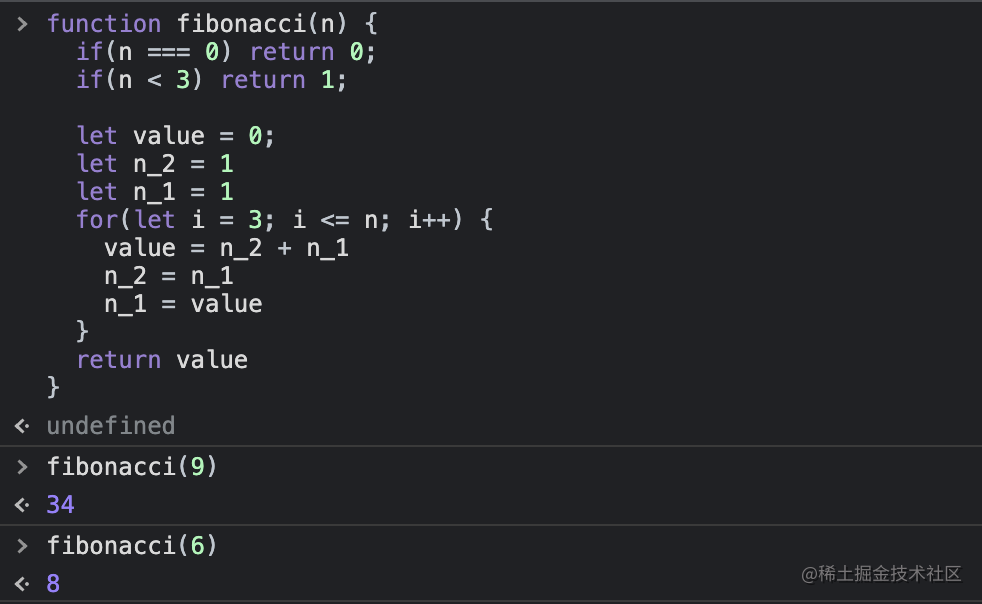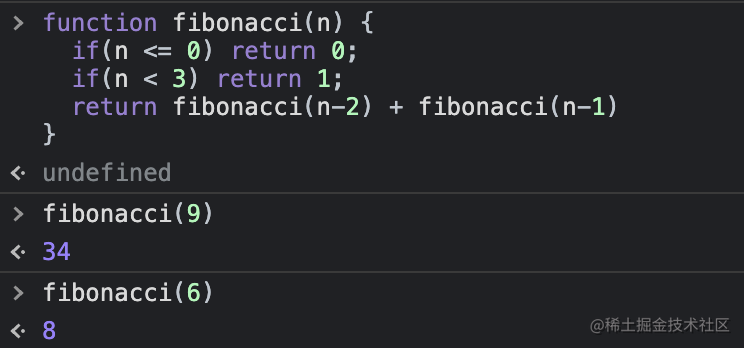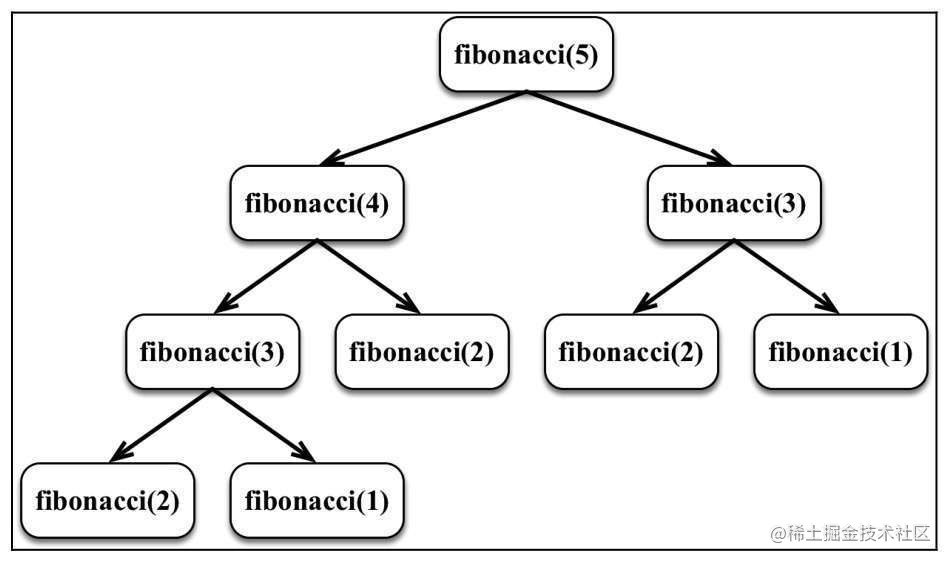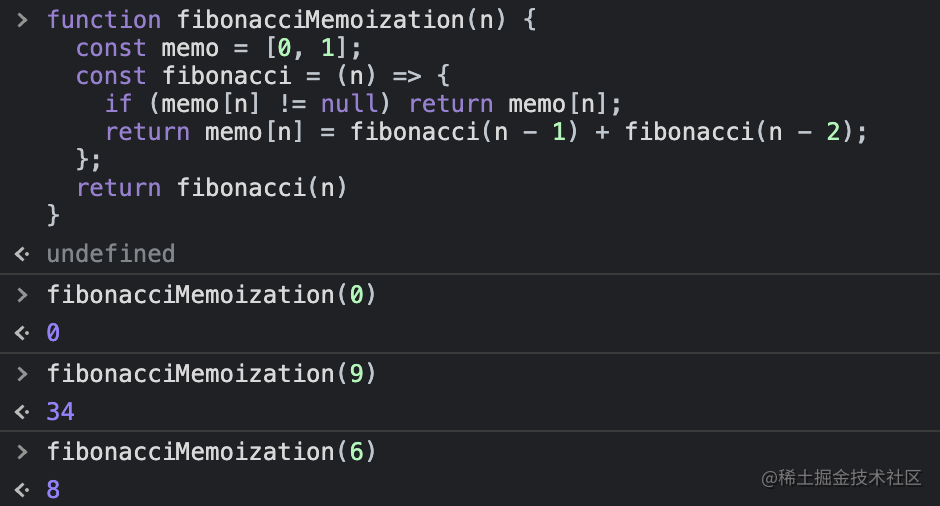# 斐波那契数列

## 斐波那契数列

```function fibonacci(n) {  if (n === 0) return 0;  if (n <= 2) return 1;
let value = 0;  let n_2 = 1;  let n_1 = 1;  for (let i = 3; i <= n; i++) {    value = n_2 + n_1;    n_2 = n_1;    n_1 = value;  }  return value;}```## 递归实现斐波那契数列

1. 最小粒度的表达式
2. 终止条件

`f(n) = f(n-2) + f(n-1)`

`function fibonacci(n) {  if (n <= 0) return 0;  if (n <= 2) return 1;  return fibonacci(n - 2) + fibonacci(n - 1);}`## 记忆化斐波那契数

`function fibonacciMemoization(n) {  const memo = [0, 1];  const fibonacci = (n) => {    if (memo[n] != null) return memo[n];    return (memo[n] = fibonacci(n - 1) + fibonacci(n - 2));  };  return fibonacci(n);}`